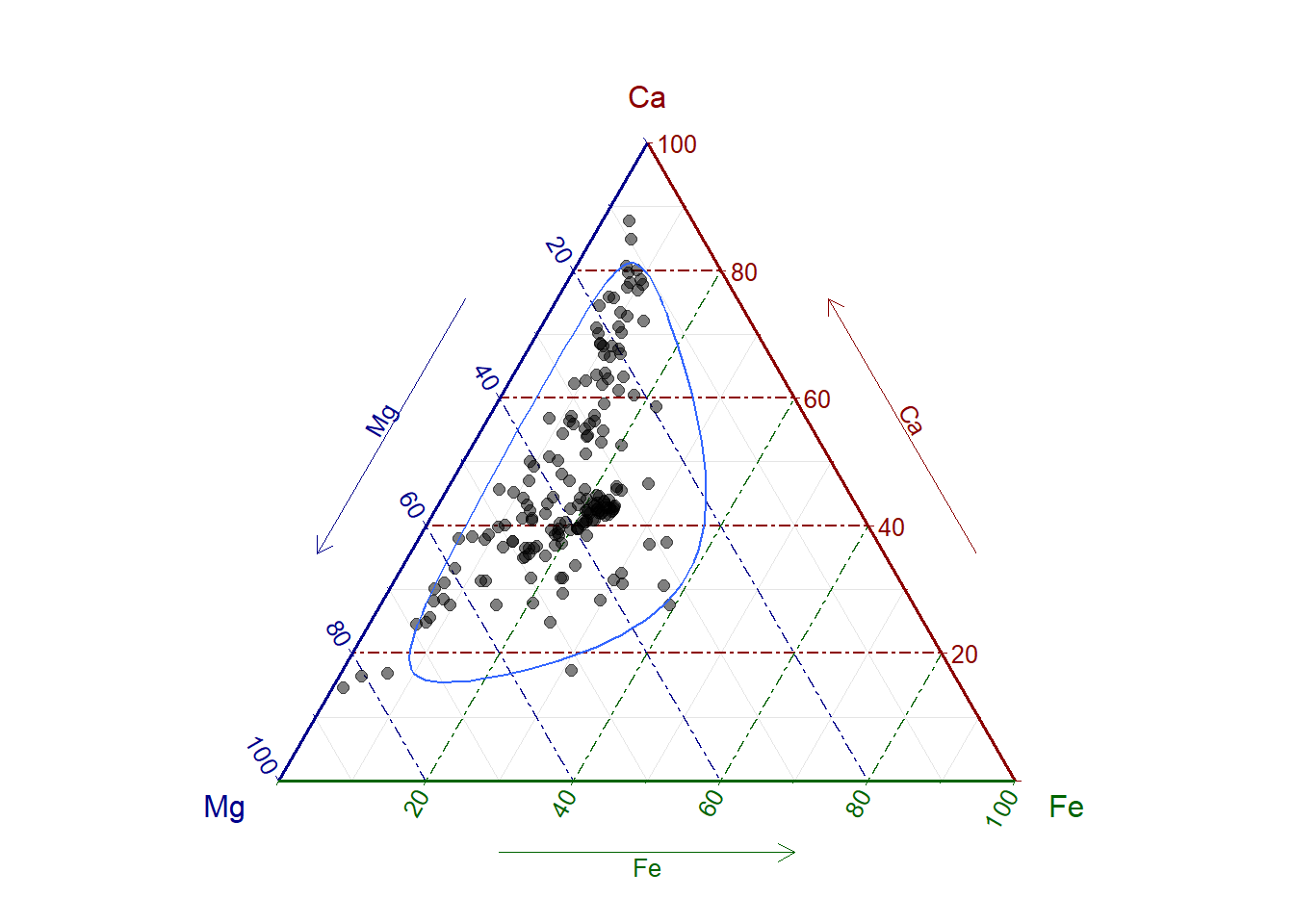ggtern is an extension to ggplot2 provided by Nicholas Hamilton specifically for the plotting of ternary diagrams. Ternary diagrams plot three variables as part of a composition and are commonly used within the fields of chemistry, petrology, mineralogy, metallurgy, materials-science, genetics and game-theory, among others. They represent a 2D simplex of a 3D (sub-)composition. For further information on the treatment of compositional data for statistical analysis take a look here.

Given some familiarity with the syntax of ggplot2 it is quite easy to produce publication quality ternary diagrams.

library(ggtern)

# data preparation
sep = "\t",
row.names = "Sample"
)
colnames(g36) <- gsub(".mg.g", "", colnames(g36))
data <- as.data.frame(g36[c("Mg", "Ca", "Fe")])

# plotting
ggtern(data = data, aes(Mg, Ca, Fe)) +
geom_point(
alpha = 0.5,
size = 2,
color = "black"
) +
theme_rgbw() + geom_confidence_tern(breaks = 0.95)Check out the webpage for more information and more examples of ternary diagrams using this great library.